• 本书全面介绍了近10年来发展的基于几何计算机视觉计算方法及其数学基础。除了上述内容外，其中摄像机视图几何及其计算方法，值得读者关注。这是因为当前计算机的性能价格比大大提高，使人们有条件在视觉系统...计算机视觉
• 本书涵盖了摄像机投影矩阵、基本矩阵和三焦点张量的几何原理、和它们的代数表达，并配有实际的例子，如它们在由幅图像进行景物重构的应用。计算机视觉
• 计算机视觉中视图几何（中文版）200-484，计算机视觉中视图几何（中文版）200-484，计算机视觉中视图几何（中文版）200-484计算机视觉
• 计算机视觉中多视几何 书籍 哈特利等 图像处理以及计算机视觉相关计算机视觉
• 从道客巴巴3000积分下载的，英文原版计算机视觉中视图几何（英文原版）计算机视觉
• 计算机视觉中视图几何-中文版本，适合对计算机视觉方向的学生学习。计算机视觉
• 计算机视觉中视图几何(中文版)。计算机视觉中视图几何(中文版)计算机视觉中
• 本质上两幅图之间的对极几何是图像平面与以基线为轴的平面束的交的几何，这种几何被广泛同于双目成像原理 如图所示，摄像机由相机中心C,C’以及各自的成像平面表示，对于任意一个空间的点X,在两个像平面上的点...

一、基础矩阵原理介绍

1、对极几何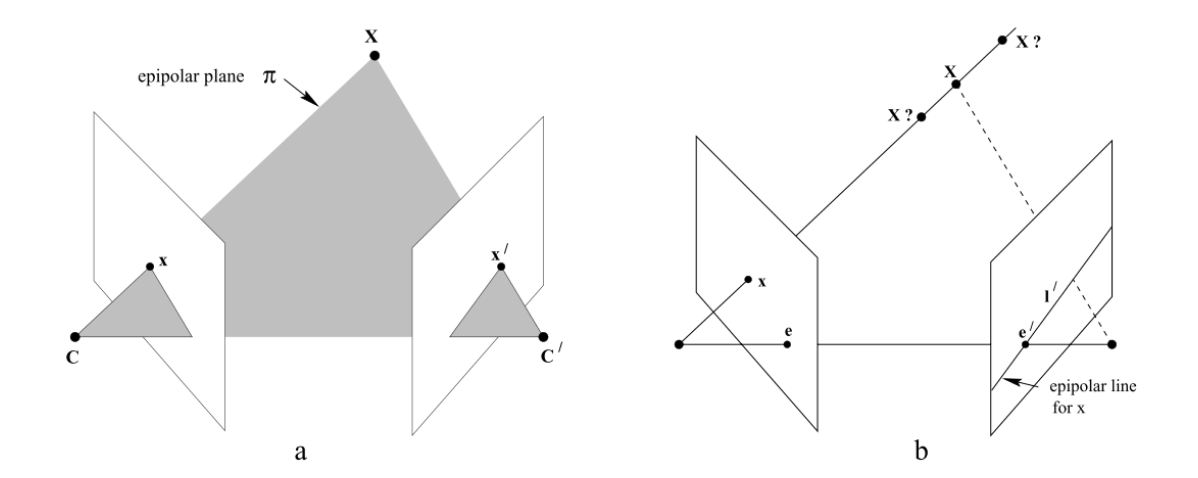本质上两幅图之间的对极几何是图像平面与以基线为轴的平面束的交的几何，这种几何被广泛同于双目成像原理中
如图所示，摄像机由相机中心C,C’以及各自的成像平面表示，对于任意一个空间中的点X,在两个像平面上的点分别为x,x’，第一幅图像上的点x反向投影成空间三维的一条射线，它由摄像机中心和x确定，这条射线向第二个图像平面上投影得到一条直线l’，显然x的投影x’必然在l’上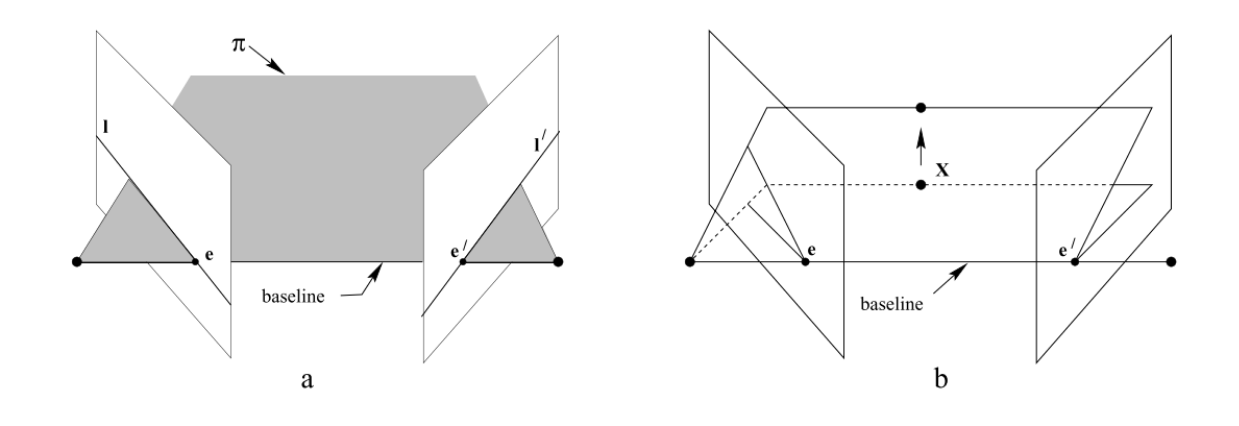如图所示，我们把相机中心的连线叫做基线(baseline),基线与两幅图像平面交于对极点e和e’，任何一条包含基线的平面pi是一张对极平面，分别与图像平面相交于l和l’，实际上，当三维位置X变化时，对应的实际上是该对极平面绕基线”旋转”，这个旋转得到的平面簇叫做对极平面束，由所有对极平面和成像平面相交得到的对极限相交于对极点
（1）对极几何的几个相关概念
对极平面束（epipolar pencil）：以基线为轴的平面束；下图给出了包含两个平面的对极平面束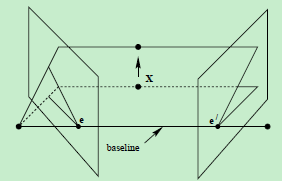对极平面（epipolar plane）：任何包含基线的平面都称为对极平面，或者说是对极平面束中的平面；例如，下图中的平面ππ就是一个对极平面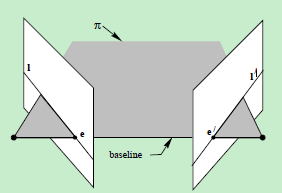对极点（epipole）：摄像机的基线与每幅图像的交点；即上图中的点e和e’

对极线（epipolar line）：对极平面与图像的交线；例如，上图中的直线l和l’

2、基础矩阵

基础矩阵是对极几何的代数表达方式
本质矩阵描述了空间中的点在两个图像的投影的对应关系
（图像中任意对应点 x↔x’ 之间的约束关系）

F 为 3x3 矩阵，秩为2，对任意匹配点对 x↔x’ 均满足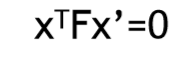基础矩阵F表示的就是这种从点到直线的映射。

（1）F的代数推导

设以第一个相机作为坐标系三维空间的点P=[X,Y,Z]，其在两个相机的像点分别为p1,p2。由于第一个相机的中心作为世界坐标系的原点，也就是说第一个相机没有旋转和平移，通过小孔相机模型可得：
p1=KP，p2=K(RP+t)

其中,K是相机的内参，R,t是第二个相机相对于第一个相机的旋转和平移。
从p1=KP可以得到P=K−1p1，带入第二个式子可得到：
p2=K(RK−1p1+t)

两边同时左乘K−1，得到
K−1p2=RK−1p1+t

设x1=K−1p1,x2=K−1p2，代入
x2=Rx1+t

两边同时左乘向量t的反对称矩阵t×,由于t×t=0，消除t
t×x2=t×Rx1

两边再同时左乘xT2
xT2t×x2=xT2t×Rx1

由于t×x2是向量t和向量x2的叉积，同时垂直于向量t和向量x2，所以左边xT2t×x2=0，得到
xT2t×Rx1=0

再将x1,x2换掉
pT2K−Tt×RK−1p1=0

上式是对极约束的另一种表示，该式子中仅包含像点，相机的旋转和平移，中间的矩阵就是基础矩阵F。
pT2Fp1=0,其中F=K−Tt×RK−1

式子F=K−Tt×RK−1，可知假如相机的内参数K是已知的，提取中间的矩阵可到
E=t×R

E被称为本质矩阵，其和基础矩阵相差相机的内参K。

（2） 基础矩阵的性质：

1、转置对称性：如果 F 是一对影像 (P,P′) 的基础矩阵（即 x′Fx=0 ），反过来 (P′,P) 的基础矩阵是 FT。证明很简单，直接对 x′Fx=0 两侧分别转置，得到 xTFTx′=0 。

2、核线：对于左影像上任意一点 x ，其在右影像上的核线为 l′=Fx 。

3、核点：任何核线都会经过核点，所以有对于左影像上任意一点 x ，e′Tl′=e′T(Fx)=0 ，于是有 e′TF=0 。同理有 Fe=0 。

4、F 具有7自由度：一个 3x3 的单应矩阵，具有8个自由度，而 F 还满足/5、detF=0，所以 F 具有7个自由度。

5、F 是相关的：F 将左影像上的一点 x 投影到右影像上一条核线 l′，投影本质上是将 x 与左核点的连线 l 投影到右影像上的核线 l′ ，所以右影像上的一条核线 l′ 对应的是左影像上的一条核线 l，这种点到线的投影不可逆。

如果已知基础矩阵F，以及一个3D点在一个像面上的像素坐标p，则可以求得在另一个像面上的像素坐标p’。这个是基础矩阵的作用，可以表征两个相机的相对位置及相机内参数。

二、代码

# coding: utf-8

# In:

from PIL import Image
from numpy import *
from pylab import *
import numpy as np

# In:

from PCV.geometry import camera
from PCV.geometry import homography
from PCV.geometry import sfm
from PCV.localdescriptors import sift

# In:

im1 = array(Image.open('D:/Python36/code/data/001.jpg'))
sift.process_image('D:/Python36/code/data/001.jpg', 'im1.sift')

im2 = array(Image.open('D:/Python36/code/data/002.jpg'))
sift.process_image('D:/Python36/code/data/002.jpg', 'im2.sift')

# In:

# In:

matches = sift.match_twosided(d1, d2)

# In:

ndx = matches.nonzero()
x1 = homography.make_homog(l1[ndx, :2].T)
ndx2 = [int(matches[i]) for i in ndx]
x2 = homography.make_homog(l2[ndx2, :2].T)

d1n = d1[ndx]
d2n = d2[ndx2]
x1n = x1.copy()
x2n = x2.copy()

# In:

figure(figsize=(16,16))
sift.plot_matches(im1, im2, l1, l2, matches, True)
show()

# In:

#def F_from_ransac(x1, x2, model, maxiter=5000, match_threshold=1e-6):
def F_from_ransac(x1, x2, model, maxiter=5000, match_threshold=1e-6):
""" Robust estimation of a fundamental matrix F from point
correspondences using RANSAC (ransac.py from
http://www.scipy.org/Cookbook/RANSAC).

input: x1, x2 (3*n arrays) points in hom. coordinates. """

from PCV.tools import ransac
data = np.vstack((x1, x2))
d = 10 # 20 is the original
# compute F and return with inlier index
F, ransac_data = ransac.ransac(data.T, model,
8, maxiter, match_threshold, d, return_all=True)
return F, ransac_data['inliers']

# In:

# find F through RANSAC
model = sfm.RansacModel()
F, inliers = F_from_ransac(x1n, x2n, model, maxiter=5000, match_threshold=1e-5)
print(F)

# In:

P1 = array([[1, 0, 0, 0], [0, 1, 0, 0], [0, 0, 1, 0]])
P2 = sfm.compute_P_from_fundamental(F)

# In:

print(P2)
print (F)

# In:

# P2, F (1e-4, d=20)
# [[ -1.48067422e+00   1.14802177e+01   5.62878044e+02   4.74418238e+03]
#  [  1.24802182e+01  -9.67640761e+01  -4.74418113e+03   5.62856097e+02]
#  [  2.16588305e-02   3.69220292e-03  -1.04831621e+02   1.00000000e+00]]
# [[ -1.14890281e-07   4.55171451e-06  -2.63063628e-03]
#  [ -1.26569570e-06   6.28095242e-07   2.03963649e-02]
#  [  1.25746499e-03  -2.19476910e-02   1.00000000e+00]]

# In:

# triangulate inliers and remove points not in front of both cameras
X = sfm.triangulate(x1n[:, inliers], x2n[:, inliers], P1, P2)

# In:

# plot the projection of X
cam1 = camera.Camera(P1)
cam2 = camera.Camera(P2)
x1p = cam1.project(X)
x2p = cam2.project(X)

# In:

figure(figsize=(16, 16))
imj = sift.appendimages(im1, im2)
imj = vstack((imj, imj))

imshow(imj)

cols1 = im1.shape
rows1 = im1.shape
for i in range(len(x1p)):
if (0<= x1p[i]<cols1) and (0<= x2p[i]<cols1) and (0<=x1p[i]<rows1) and (0<=x2p[i]<rows1):
plot([x1p[i], x2p[i]+cols1],[x1p[i], x2p[i]],'c')
axis('off')
show()

# In:

d1p = d1n[inliers]
d2p = d2n[inliers]

# In:

im3 = array(Image.open('D:/Python36/code/data/003.jpg'))
sift.process_image('D:/Python36/code/data/003.jpg', 'im3.sift')

# In:

matches13 = sift.match_twosided(d1p, d3)

# In:

ndx_13 = matches13.nonzero()
x1_13 = homography.make_homog(x1p[:, ndx_13])
ndx2_13 = [int(matches13[i]) for i in ndx_13]
x3_13 = homography.make_homog(l3[ndx2_13, :2].T)

# In:

figure(figsize=(16, 16))
imj = sift.appendimages(im1, im3)
imj = vstack((imj, imj))

imshow(imj)

cols1 = im1.shape
rows1 = im1.shape
for i in range(len(x1_13)):
if (0<= x1_13[i]<cols1) and (0<= x3_13[i]<cols1) and (0<=x1_13[i]<rows1) and (0<=x3_13[i]<rows1):
plot([x1_13[i], x3_13[i]+cols1],[x1_13[i], x3_13[i]],'c')
axis('off')
show()

# In:

P3 = sfm.compute_P(x3_13, X[:, ndx_13])

# In:

print(P3)

# In:

print(P1)
print(P2)
print(P3)

# In:

# Can't tell the camera position because there's no calibration matrix (K)

三、实验结果

1、室外场景

1）、运行结果

|| 第一张图和第二张图的sift特征点匹配图：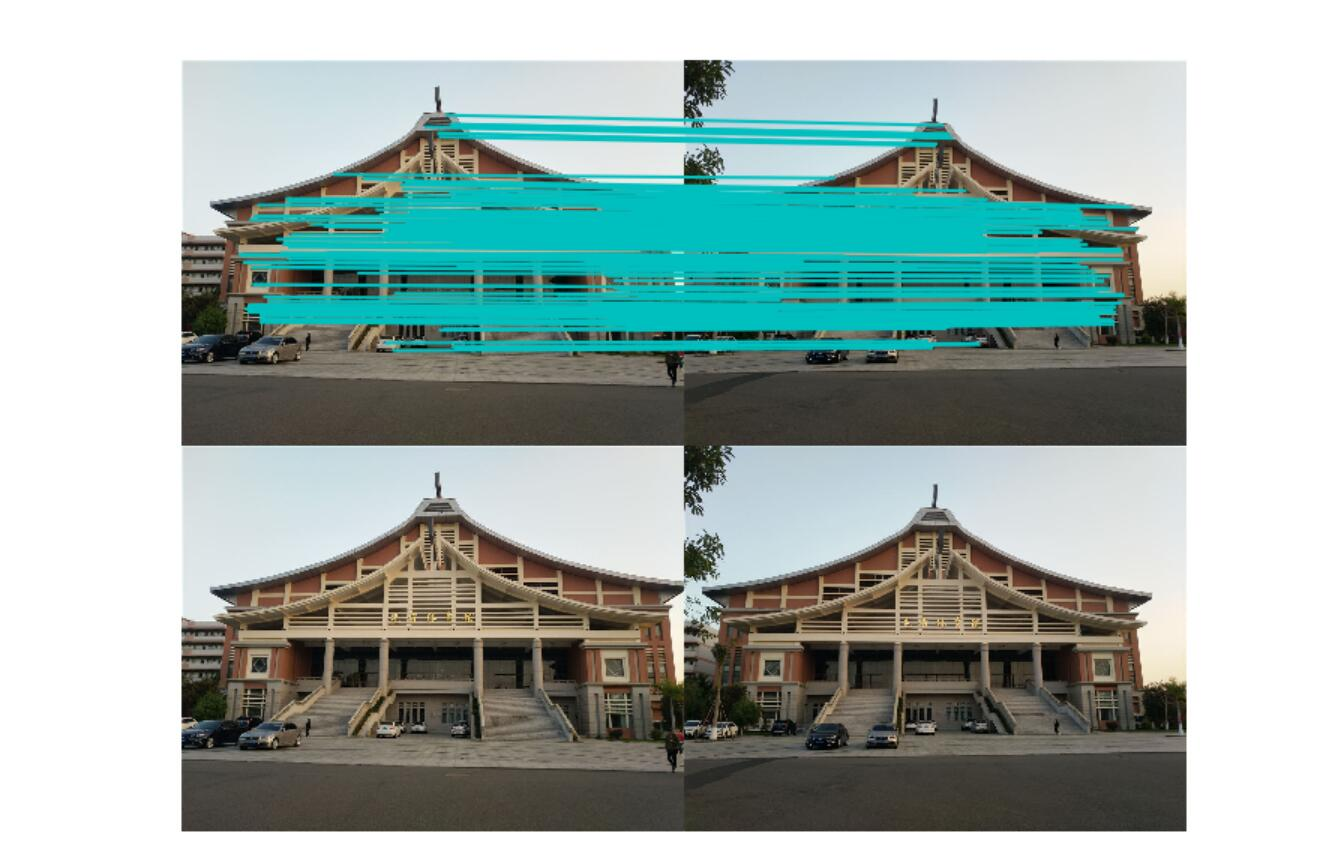------------------------------------------图3-1-1------------------------------------------

|| 经过ransac算法筛选得到的特征点匹配图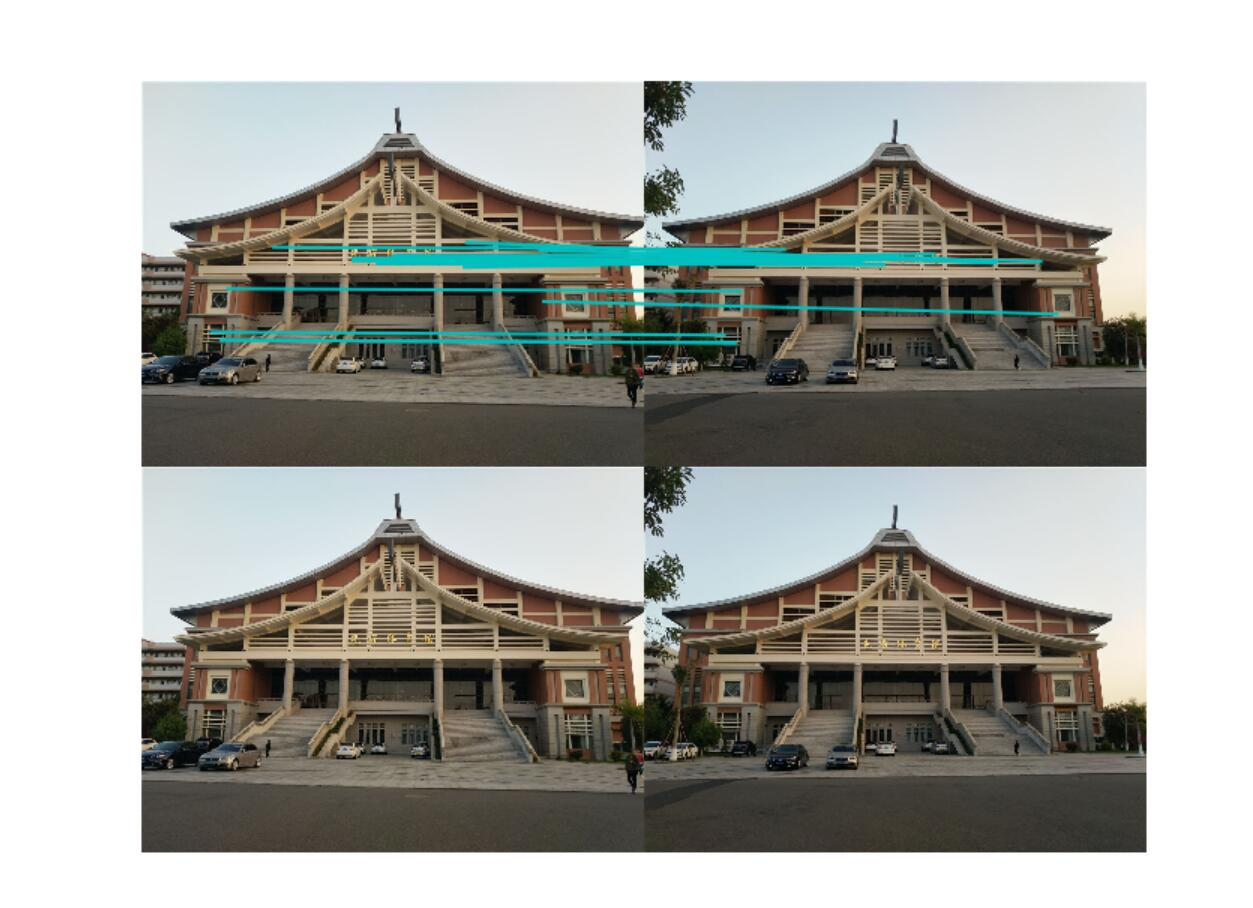------------------------------------------图3-1-2------------------------------------------

|| 经过前面的得到的基础矩阵对第一张图和第三张图进行特征匹配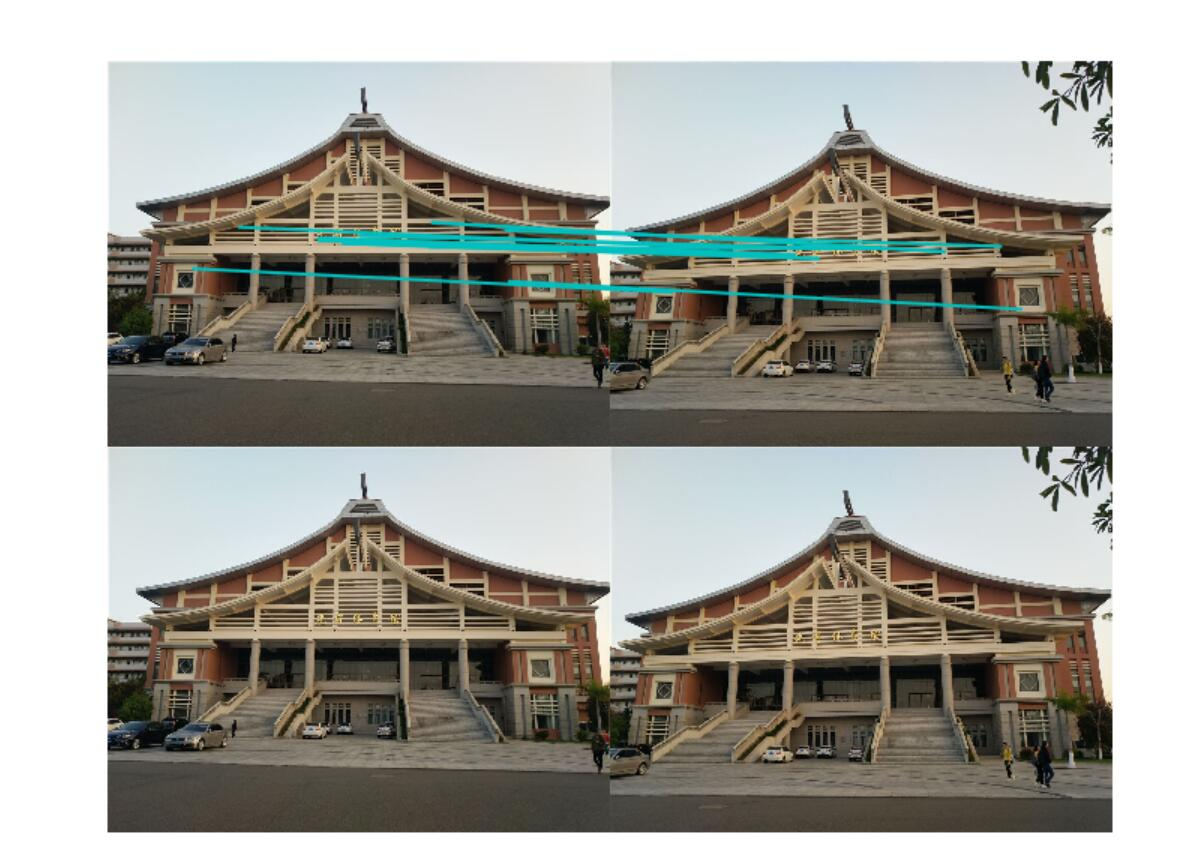------------------------------------------图3-1-3------------------------------------------

2）、基础矩阵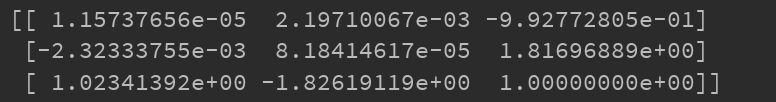------------------------------------------图3-1-4------------------------------------------

2、室内场景

1）、运行结果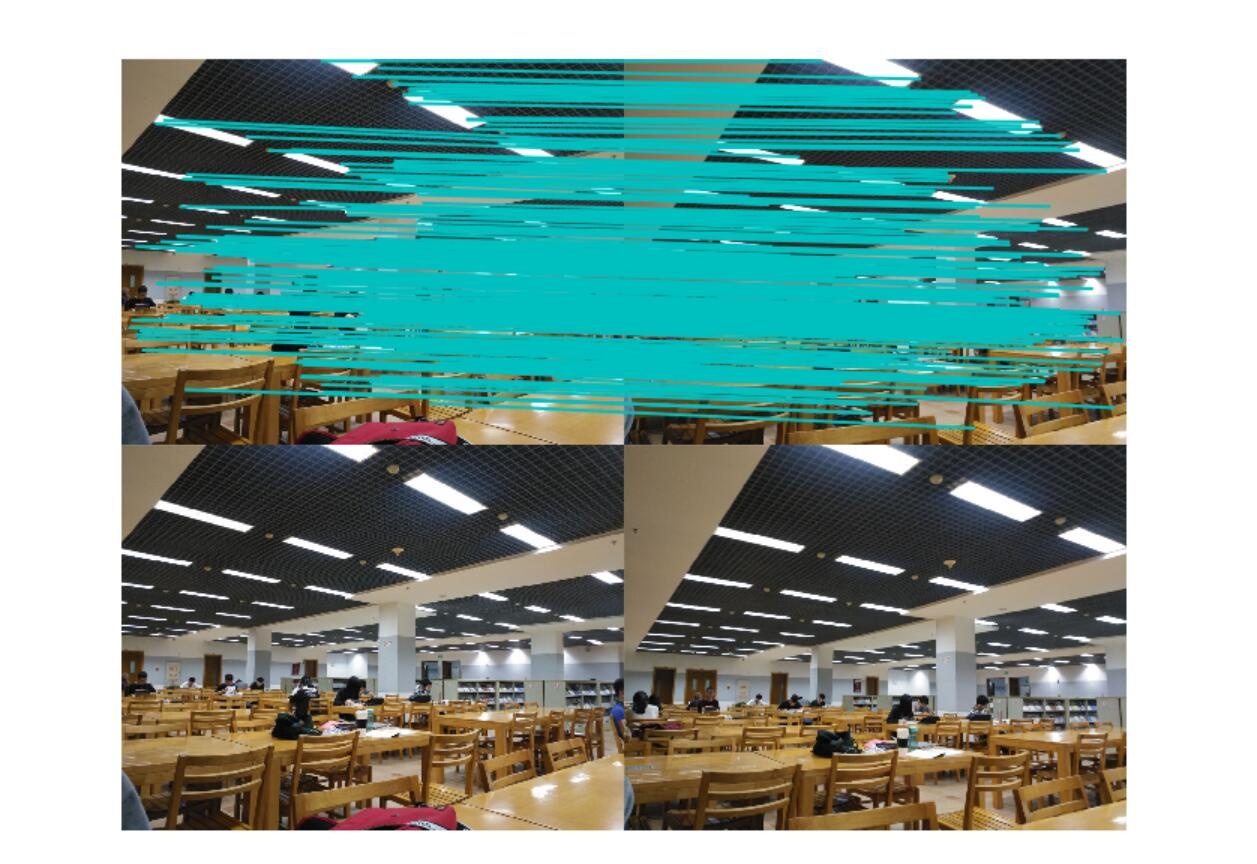------------------------------------------图3-2-1------------------------------------------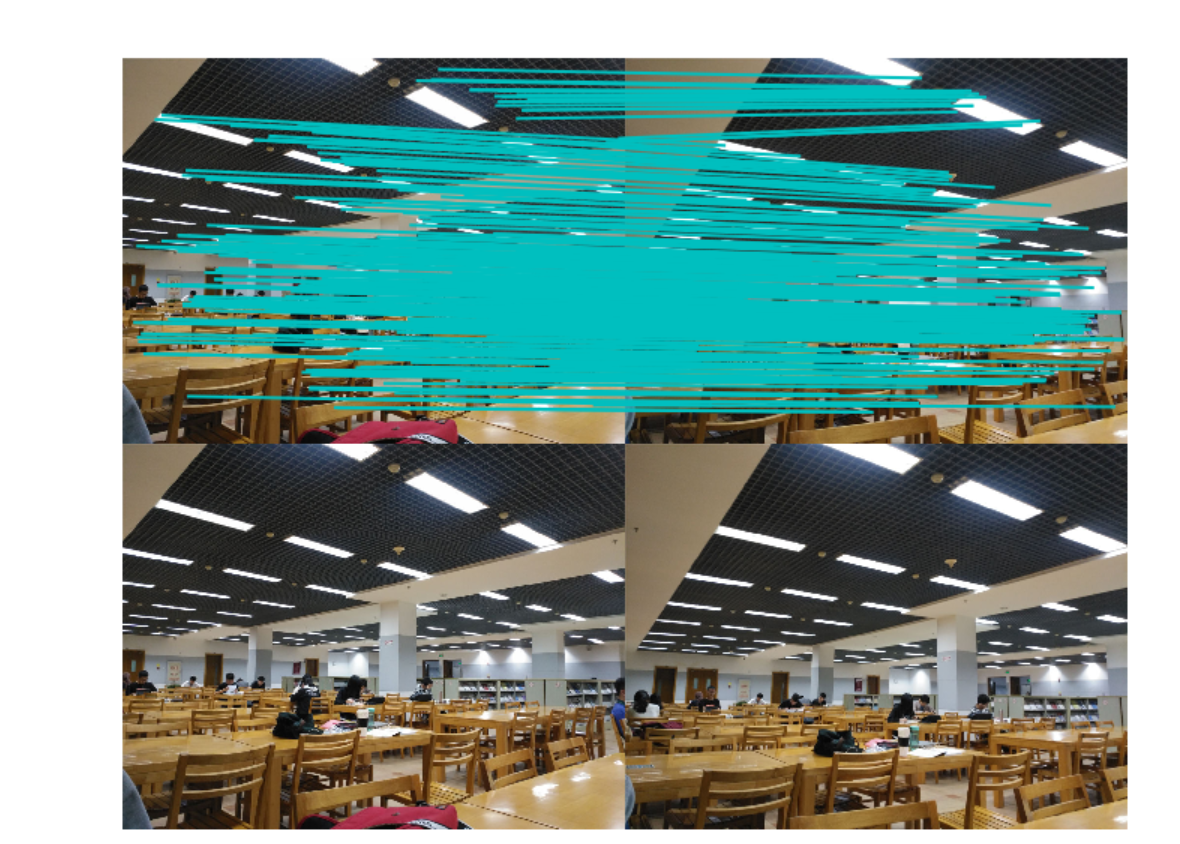------------------------------------------图3-2-2------------------------------------------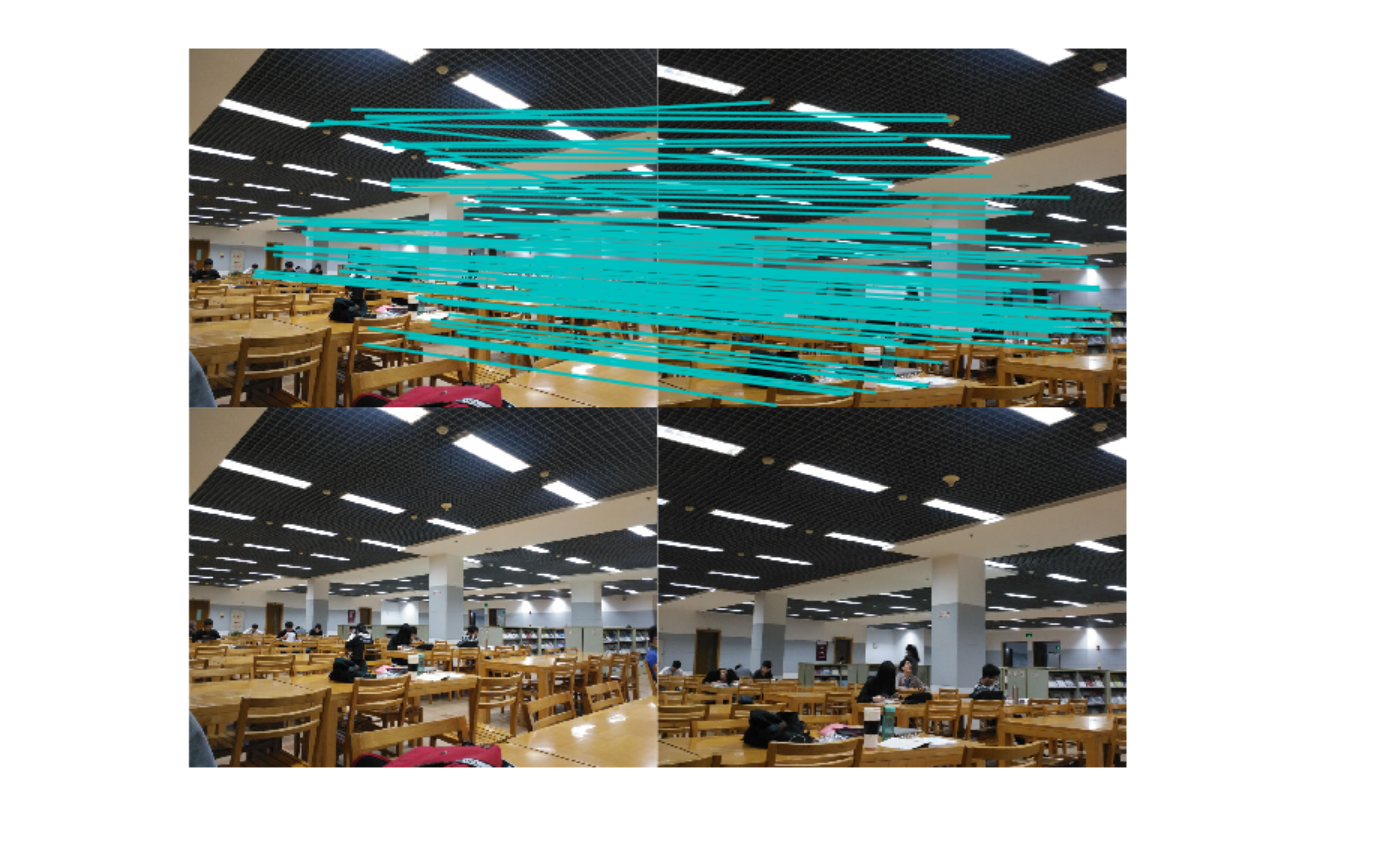------------------------------------------图3-2-3------------------------------------------

2）、基础矩阵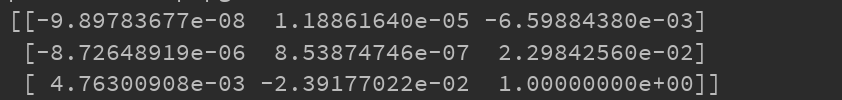三、遇到问题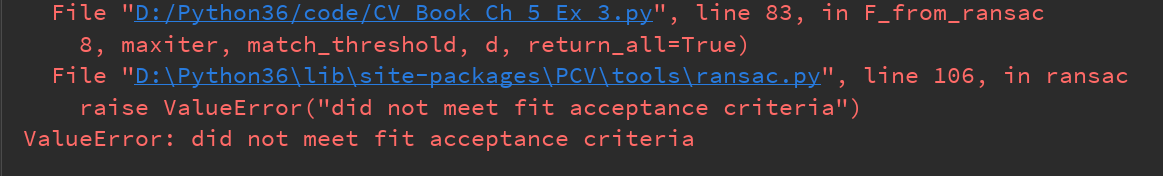在实际图像中获取到的数据，常常会包含有噪声数据，这些噪声数据会使对模型的构建造成干扰，我们称这样的噪声数据点为outliers，那些对于模型构建起积极作用的我们称它们为inliers，RANSAC做的一件事就是先随机的选取一些点，用这些点去获得一个模型(这个讲得有点玄，如果是在做直线拟合的话，这个所谓的模型其实就是斜率)，然后用此模型去测试剩余的点，如果测试的数据点在误差允许的范围内，则将该数据点判为inlier，否则判为outlier。inliers的数目如果达到了某个设定的阈值，则说明此次选取的这些数据点集达到了可以接受的程度，否则继续前面的随机选取点集后所有的步骤，不断重复此过程，直到找到选取的这些数据点集达到了可以接受的程度为止，此时得到的模型便可认为是对数据点的最优模型构建。

解决：修改阈值，使得inliers的数目多一些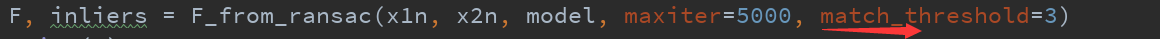注意：拍摄角度很重要，很有可能拍出来的照片找不到匹配点。

展开全文• 本书涵盖了摄像机投影矩阵、基本矩阵和三焦点张量的几何原理、和它们的代数表达，并配有实际的例子，如它们在由幅图像进行景物重构的应用。
• 计算机视觉中视图几何》，第2版，韦穗、章权兵 译 Page 44， 例2.28上面5行 在极限情形下，x将在C上，其极线与C有二阶接触点x，于是得到 “二阶接触点”是什么意思？ 2021年9月6日。 Page 55， 3.2.4上面2行 ...

《计算机视觉中的多视图几何》，第2版，韦穗、章权兵 译

Page 41， 倒数第5行

C ∞ ∗ C^{*}_{\infty} 已辨认的摄影框架下，按式(2.22)， c o s α cos \alpha c o s β cos \beta 可由 l ′ = a ′ × b ′ l'=a'\times b' m ′ = c ′ × a ′ m'=c'\times a' n ′ = b ′ × c ′ n'=b'\times c' 计算出来。

Page 44， 例2.28上面5行

在极限情形下，x将在C上，其极线与C有二阶接触点x，于是得到

“二阶接触点”是什么意思？ 2021年9月6日。

Page 55， 3.2.4上面2行

对偶二次曲面的成像的代数比点二次曲面的简单得多。

“成像的代数…简单得多”是什么意思？2021年9月6日。

Page 164， 图8.13

图标（a）和（b）应该是标反了。

Page 171， 算法8.1

（3）将像直线 l 1 l_1 上的四个点 b 2 b_2 t 1 ~ \tilde{t_1} t 2 t_2 v v 分别用…
应该是直线 l 2 l_2

Page 283， 第4行

…从而可不必使用一些（可能）不熟悉的符号…

是不是这个意思 “从而避免使用一些（可能）不熟悉的符号”。

展开全文• 计算机视觉中视图几何-+第一版+中文 PDF文档 带目录
• 计算机视觉中视图几何-第一版-中文完整版，非常清晰的版本，快来下载吧。多视几何
• 国外计算机视觉领域经典教材《计算机视觉中视角几何》全套PPT，希望对大家学习CV有所帮助！
• 1. 单视几何（应用 单幅图像测量） 2. 两视几何（Epipolar Geometry 约束） 空间平面与Homography 3. 三视几何（Trifocal Geometry 约束） 算机视觉多视几何，有需要的请下载。
• 计算机视觉——多视图几何

千次阅读 2020-04-21 23:41:10
视图几何1.前言1.1 视图几何概念2. 基本原理2.1 对极几何2.2 基础矩阵2.2.1 基础矩阵推导2.2.2 求解基础矩阵3. 实验过程3.1 实验数据准备3.2 实验代码3.3 实验结果及分析4. 实验遇到的问题 1.前言 1.1 视图...

1.前言

1.1 多视图几何概念

多视图几何是利用在不同视点所拍摄图像间的关系，来研究照相机之间或者特征之间关系的一门科学。

多视图几何（Multiple View Geometry）多视图几何主要研究用几何的方法，通过若干幅二维图像，来恢复三维物体。换言之就是研究三维重构，主要应用与计算机视觉中。

三维重构，即如何从静止物体不同视点下的图像中恢复物体三维几何结构信息。

在三维重构的过程中摄像机标定是一个极其重要环节，摄像机标定的研究分为三个部分：
（1）基于场景结构信息的标定；
（2）基于摄像机主动信息（纯旋转）的自标定；
（3）不依赖场景结构和摄像机主动信息的自标定。

2. 基本原理

2.1 对极几何

对极几何（Epipolar Geometry）描述的是两幅视图之间的内在射影关系，与外部场景无关，只依赖于摄像机内参数和这两幅试图之间的的相对姿态。

如下图所示，X为空间中的一点，x和x’为X在不同像平面中的投影，其中X与C, C’, x, x’ 共面。

tips：如果知道空间中点X在一幅图像中的投影点x，是无法确定空间中点X的位置以及X在其他图像中的投影点。

这里可以明确如下概念：

对极平面束（epipolar pencil）：以基线为轴的平面束；下图给出了包含两个平面的对极平面束。

对极平面（epipolar plane）：任何包含基线的平面都称为对极平面，或者说是对极平面束中的平面；例如，下图中的平面π就是一个对极平面。

对极点（epipole）：摄像机的基线与每幅图像的交点；即下图中的点e和e’。

对极线（epipolar line）：对极平面与图像的交线；例如，上图中的直线l和l’。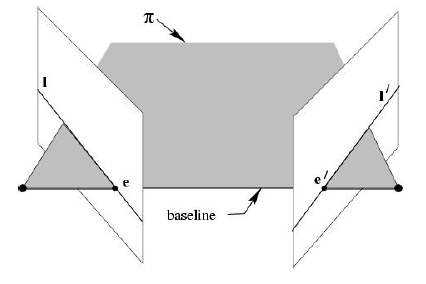点x、x’与摄像机中心C和C’是共面的，并且与空间点X也是共面的，这5个点共面于平面π，这是一个最本质的约束，即5个点决定了一个平面π。

由该约束，可以推导出一个重要性质：由图像点x和x’反投影的射线共面，并且，在平面π上。 在搜索点对应中，该性质非常重要
由此引出基础矩阵的概念。

2.2.1 基础矩阵推导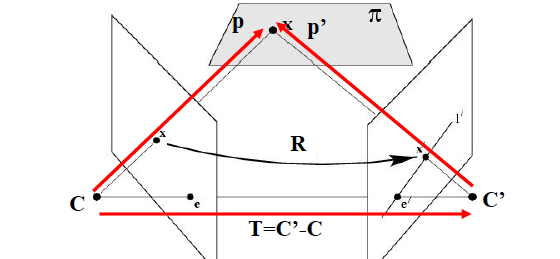如上图所示，设X分别以C,C’为坐标原点，x的坐标被表示为p和p’，则有
p = R p ′ + T p=Rp'+T
其中R、T表示在两个坐标系之间的旋转和位移。
在以C为坐标系原点的时候有： x = K p ⇒ p = K − 1 x x=Kp \Rightarrow p=K^{-1}x
在以C’为坐标系原点的时候有： x ′ = K ′ p ′ ⇒ p ′ = K ′ − 1 x ′ x'=K'p' \Rightarrow p'=K'^{-1}x'
根据三点共面的性质可以知道：
( p − T ) T ( T × p ) = 0 (p-T)^T(T×p)=0
又因为 p = R p ′ + T p=Rp'+T 得到 p − T = R p ′ p-T=Rp' ，所以上式可化简如下：
( R T p ′ ) T ( T × p ) = 0 (R^Tp')^T(T×p)=0
其中 T × p = S p T×p=Sp ，又 S = [ 0 − T z T y T z 0 − T x − T y T x 0 ] S=\begin{bmatrix} 0& -T_z & T_y\\ T_z& 0 &-T_x \\ -T_y& T_x & 0 \end{bmatrix}
所以 ( R T p ′ ) T ( T × p ) = 0 (R^Tp')^T(T×p)=0 可写为下式：
( R T p ′ ) T ( S p ) = 0 ⇒ ( p ′ T R ) ( S p ) = 0 ⇒ p ′ T E p = 0 (R^Tp')^T(Sp)=0\Rightarrow (p'^TR)(Sp)=0\Rightarrow p'^TEp=0
最终得到的如上式为本质矩阵。本质矩阵描述了空间中的点X在两个坐标系中的坐标对应关系。

根据上一篇博客中提到的 K K K ′ K' 分别为两个相机的内参矩阵，有：
p = K − 1 x p ′ = K ′ − 1 x ′ p=K^{-1}x \quad \quad p'=K'^{-1}x'
用上式代替 p ′ T E p = 0 p'^TEp=0 中的p和p’ 可以得到下式：
( K − 1 x ) T E ( K ′ − 1 x ′ ) = 0 (K^{-1}x)^TE(K'^{-1}x')=0

⇒ x ′ T K − T E K − 1 x = 0 \Rightarrow x'^TK^{-T}EK^{-1}x=0

⇒ x ′ T F x = 0 \Rightarrow x'^TFx=0
经推到得到了上述基础矩阵 F F ,基础矩阵描述了空间中的点在两个像平面中的坐标对应关系。

注意：
分别以C,C’为坐标原点，x的坐标被表示为p和p’，则有下式：
p ′ T E p = 0 p'^TEp=0
其实E表示p’和p之间的位置关系，E为本质矩阵。

2.2.2 求解基础矩阵

基本矩阵是由下述方程定义：
x ′ T F x = 0 x'^TFx=0
其中 x ↔ x ′ x ↔ x ′ x↔x'x↔x′ 是两幅图像的任意一对匹配点。由于每一组点的匹配提供了计算 F F 系数的一个线性方程，当给定至少7个点（3×3的齐次矩阵减去一个尺度，以及一个秩为2的约束），方程就可以计算出未知的 F F
记点的坐标为 x = ( x , y , 1 ) T ， x ′ = ( x ′ , y ′ , 1 ) T ， x=(x,y,1)^T，x'=(x',y',1)^T， 则对应的方程为:
[ x x 1 ] [ f 11 f 12 f 13 f 21 f 22 f 23 f 31 f 32 f 33 ] [ x ′ y ′ 1 ] = 0 \begin{bmatrix} x& x& 1 \end{bmatrix}\begin{bmatrix} f_{11}& f_{12}& f_{13}\\ f_{21}& f_{22}& f_{23}\\ f_{31}& f_{32}& f_{33}\\ \end{bmatrix}\begin{bmatrix} x'\\ y'\\ 1 \end{bmatrix}=0
展开后有：
x ′ x f 11 + x ′ y f 12 + x ′ f 13 + y ′ x f 21 + y ′ y f 22 + y ′ f 23 + x f 31 + y f 32 + f 33 = 0 x'xf_{11}+x'yf_{12}+x'f_{13}+y'xf_{21}+y'yf_{22}+y'f_{23}+xf_{31}+yf_{32}+f_{33}=0
把矩阵 F F 写成列向量的形式，则有：
给定 n n 组点的集合，我们有如下方程：
A f = [ x 1 ′ x 1 x 1 ′ y 1 x 1 ′ y 1 ′ x 1 y 1 ′ y 1 y 1 ′ x 1 y 1 1 ⋮ ⋮ ⋮ ⋮ ⋮ ⋮ ⋮ ⋮ ⋮ x n ′ x n x n ′ y n x n ′ y n ′ x n y n ′ y n y n ′ x n y n 1 ] f = 0 Af=\begin{bmatrix} x_1'x_1& x_1'y_1&x_1' & y_1'x_1& y_1'y_1& y_1' & x_1 & y_1& 1\\ ⋮ & ⋮& ⋮& ⋮& ⋮ &⋮ & ⋮& ⋮&⋮ \\ x_n'x_n &x_n'y_n &x_n' & y_n'x_n &y_n'y_n &y_n' &x_n &y_n &1 \end{bmatrix}f=0

如果存在确定（非零）解，则系数矩阵 A A 的秩最多是 8 8 。由于F是齐次矩阵，所以如果矩阵 A A 的秩为 8 8 ，则在差一个尺度因子的情况下解是唯一的。可以直接用线性算法解得。

如果由于点坐标存在噪声则矩阵 A A 的秩可能大于 8 8 （也就是等于 9 9 ，由于 A A n ∗ 9 n*9 的矩阵）。这时候就需要求最小二乘解，这里就可以用SVD来求解，ff的解就是系数矩阵 A A 最小奇异值对应的奇异向量，也就是 A A 奇异值分解后 A = U D V T A=UDV^T
中矩阵 V V 的最后一列矢量，这是在解矢量 f f 在约束 ∥ f ∥ \left \| f\right \| 下取 ∥ A f ∥ \left \|Af \right \| 最小的解。

求解基础矩阵可分为如下两步：

1. 求线性解。由系数矩阵 A A 最小奇异值对应的奇异矢量 f f 求的 F F
2. 奇异性约束。是最小化Frobenius范数 ∥ F − F ′ ∥ \left \|F-F' \right \| 的F’代替F。

8点求解基础矩阵算法是计算基本矩阵的最简单的方法。为了提高解的稳定性和精度，往往会对输入点集的坐标先进行归一化处理。

3. 实验过程

3.1 实验数据准备

在本次实验中，拍摄三组不同的图像来求解基础矩阵并且观察极线的分布，数据如下：
第一组数据：左右拍摄，极点位于图像平面上（左右拍摄）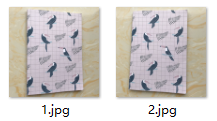第二组数据：像平面接近平行，极点位于无穷远（左右平移）第三组数据：图像拍摄位置位于前后（远近不同）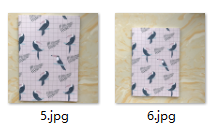3.2 基础矩阵的计算

关于基础矩阵的计算，本次实验设计7点、8点、10点计算基础矩阵，对于匹配点的个数对于基础矩阵的结果有何影响。

3.2.1 实验代码

from PIL import Image
from numpy import *
from pylab import *
import numpy as np
from PCV.geometry import camera
from PCV.geometry import homography
from PCV.geometry import sfm
from PCV.localdescriptors import sift
# 载入图像，并计算特征
im1 = array(Image.open('data/5.jpg'))
sift.process_image('data/5.jpg', 'im5.sift')
im2 = array(Image.open('data/6.jpg'))
sift.process_image('data/6.jpg', 'im6.sift')
# 匹配特征
matches = sift.match_twosided(d1, d2)
ndx = matches.nonzero()
# 使用齐次坐标表示，并使用 inv(K) 归一化
x1 = homography.make_homog(l1[ndx, :2].T)
ndx2 = [int(matches[i]) for i in ndx]
x2 = homography.make_homog(l2[ndx2, :2].T)
x1n = x1.copy()
x2n = x2.copy()
print(len(ndx))
figure(figsize=(16,16))
sift.plot_matches(im1, im2, l1, l2, matches, True)
show()
# Don't use K1, and K2
#def F_from_ransac(x1, x2, model, maxiter=5000, match_threshold=1e-6):
def F_from_ransac(x1, x2, model, maxiter=5000, match_threshold=1e-6):
""" Robust estimation of a fundamental matrix F from point
correspondences using RANSAC (ransac.py from
http://www.scipy.org/Cookbook/RANSAC).
input: x1, x2 (3*n arrays) points in hom. coordinates. """
from PCV.tools import ransac
data = np.vstack((x1, x2))
d = 20 # 20 is the original
# compute F and return with inlier index
F, ransac_data = ransac.ransac(data.T, model,8, maxiter, match_threshold, d, return_all=True)
return F, ransac_data['inliers']
# find E through RANSAC
# 使用 RANSAC 方法估计 E
model = sfm.RansacModel()
F, inliers = F_from_ransac(x1n, x2n, model, maxiter=5000, match_threshold=1e-4)
print(len(x1n))
print(len(inliers))
# 计算照相机矩阵（P2 是 4 个解的列表）
P1 = array([[1, 0, 0, 0], [0, 1, 0, 0], [0, 0, 1, 0]])
P2 = sfm.compute_P_from_fundamental(F)
# triangulate inliers and remove points not in front of both cameras
X = sfm.triangulate(x1n[:, inliers], x2n[:, inliers], P1, P2)
# plot the projection of X
cam1 = camera.Camera(P1)
cam2 = camera.Camera(P2)
x1p = cam1.project(X)
x2p = cam2.project(X)
figure()
imshow(im1)
gray()
plot(x1p, x1p, 'o')
#plot(x1, x1, 'r.')
axis('off')
figure()
imshow(im2)
gray()
plot(x2p, x2p, 'o')
#plot(x2, x2, 'r.')
axis('off')
show()
figure(figsize=(16, 16))
im3 = sift.appendimages(im1, im2)
im3 = vstack((im3, im3))
imshow(im3)
cols1 = im1.shape
rows1 = im1.shape
for i in range(len(x1p)):
if (0<= x1p[i]<cols1) and (0<= x2p[i]<cols1) and (0<=x1p[i]<rows1) and (0<=x2p[i]<rows1):
plot([x1p[i], x2p[i]+cols1],[x1p[i], x2p[i]],'c')
axis('off')
show()
print(F)
x1e = []
x2e = []
ers = []
for i,m in enumerate(matches):
if m>0: #plot([locs1[i],locs2[m]+cols1],[locs1[i],locs2[m]],'c')
x1=int(l1[i])
y1=int(l1[i])
x2=int(l2[int(m)])
y2=int(l2[int(m)])
# p1 = array([l1[i], l1[i], 1])
# p2 = array([l2[m], l2[m], 1])
p1 = array([x1, y1, 1])
p2 = array([x2, y2, 1])
# Use Sampson distance as error
Fx1 = dot(F, p1)
Fx2 = dot(F, p2)
denom = Fx1**2 + Fx1**2 + Fx2**2 + Fx2**2
e = (dot(p1.T, dot(F, p2)))**2 / denom
x1e.append([p1, p1])
x2e.append([p2, p2])
ers.append(e)
x1e = array(x1e)
x2e = array(x2e)
ers = array(ers)
indices = np.argsort(ers)
x1s = x1e[indices]
x2s = x2e[indices]
ers = ers[indices]
x1s = x1s[:20]
x2s = x2s[:20]
figure(figsize=(16, 16))
im3 = sift.appendimages(im1, im2)
im3 = vstack((im3, im3))
imshow(im3)
cols1 = im1.shape
rows1 = im1.shape
for i in range(len(x1s)):
if (0<= x1s[i]<cols1) and (0<= x2s[i]<cols1) and (0<=x1s[i]<rows1) and (0<=x2s[i]<rows1):
plot([x1s[i], x2s[i]+cols1],[x1s[i], x2s[i]],'c')
axis('off')
show()

3.2.2 实验结果及分析

① 第一组数据
七点匹配点计算基础矩阵：
控制台输出：

461
461
36
[[  1.97239734e-07  -4.26561273e-05   2.52904527e-02]
[  4.24989402e-05  -1.90178422e-06  -2.44330900e-02]
[ -2.44701559e-02   2.30334778e-02   1.00000000e+00]]

结果图：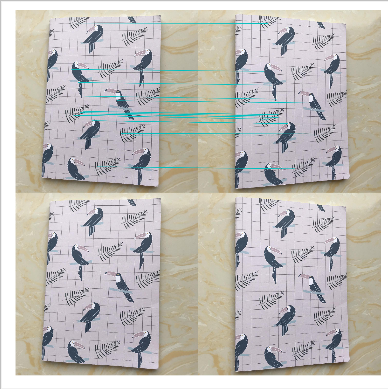八点匹配点计算基础矩阵：
控制台输出：

461
461
29
[[ -2.55224960e-07  -3.00786795e-07   1.58976498e-03]
[  2.89707522e-06   1.45441317e-07  -1.46080960e-02]
[ -2.81378730e-03   1.23999326e-02   1.00000000e+00]]

结果图：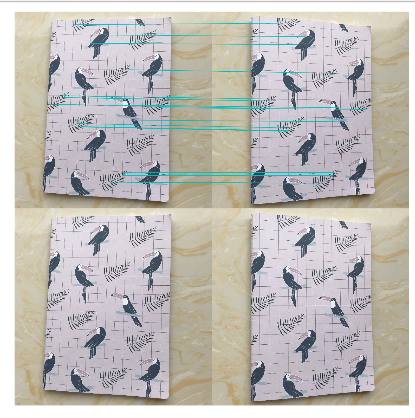十点匹配点计算基础矩阵：
控制台输出：

processed tmp.pgm to im1.sift
processed tmp.pgm to im2.sift
461
461
36
[[  6.79062229e-06  -3.58141837e-06  -3.86536085e-02]
[  4.48539039e-06  -1.53238078e-07  -5.44511839e-03]
[  2.94374668e-02   3.04050052e-03   1.00000000e+00]]

结果图：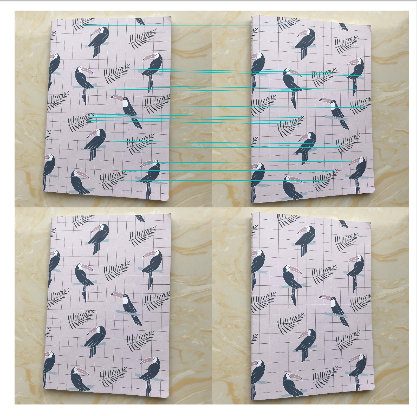② 第二组数据
七点匹配点计算基础矩阵：
控制台输出：

734
734
32
[[  4.41348662e-08  -3.42650050e-06   2.34914137e-03]
[  3.37627195e-06   3.86920082e-08  -2.77634118e-03]
[ -2.51280140e-03   1.50309243e-03   1.00000000e+00]]

结果图：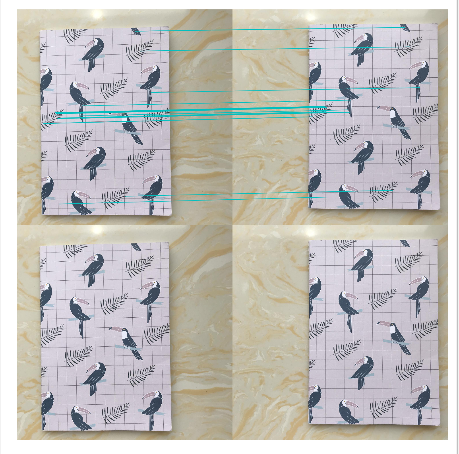八点匹配点计算基础矩阵：
控制台输出：

734
734
59
[[  5.13681399e-08  -2.72006999e-06   2.33030990e-03]
[  2.71521610e-06   1.85826640e-08  -2.25616582e-03]
[ -2.51198132e-03   1.22928879e-03   1.00000000e+00]]

结果图：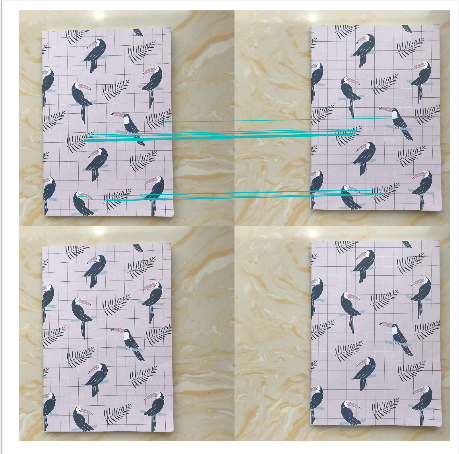十点匹配点计算基础矩阵：
控制台输出：

734
734
46
[[  3.32345027e-08  -5.21542621e-06   1.99979624e-03]
[  5.14872251e-06   2.89078827e-08  -6.53046230e-03]
[ -2.23058142e-03   4.65965814e-03   1.00000000e+00]]

结果图：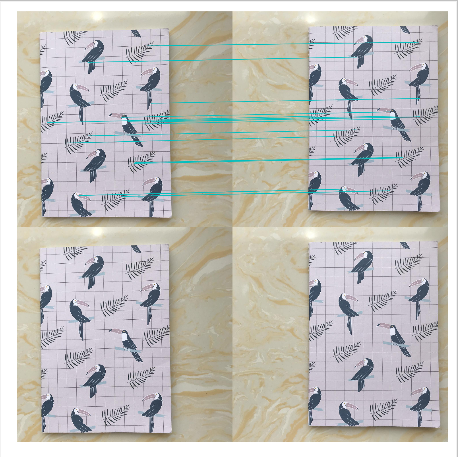③第三组数据
七点匹配点计算基础矩阵：
控制台输出：

632
632
28
[[  2.87480615e-06  -2.51191641e-04   1.83003566e-01]
[  2.49457112e-04   3.69779415e-06  -1.27817047e-01]
[ -1.69252496e-01   1.14233511e-01   1.00000000e+00]]

结果图：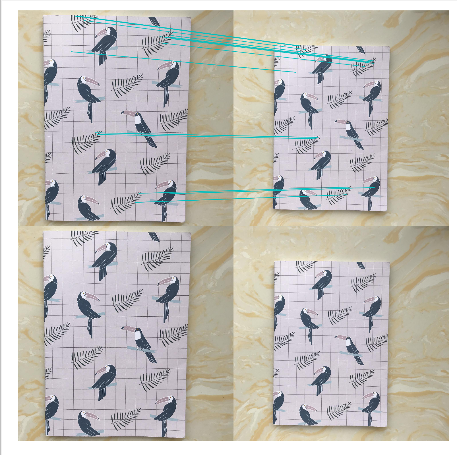八点匹配点计算基础矩阵：
控制台输出：

632
632
32
[[  3.78497574e-08   2.12332184e-05  -3.28211216e-02]
[ -2.20359610e-05   4.36071279e-07   2.63780580e-02]
[  3.62485855e-02  -2.94763915e-02   1.00000000e+00]]

结果图：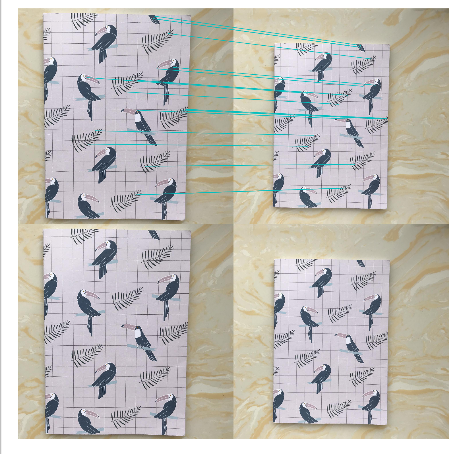十点匹配点计算基础矩阵：
控制台输出：

632
632
53
[[  4.66996990e-08  -2.04474321e-05   1.99506699e-02]
[  2.07208420e-05   2.14957900e-07  -1.06213988e-02]
[ -2.00422549e-02   9.28538206e-03   1.00000000e+00]]

结果图：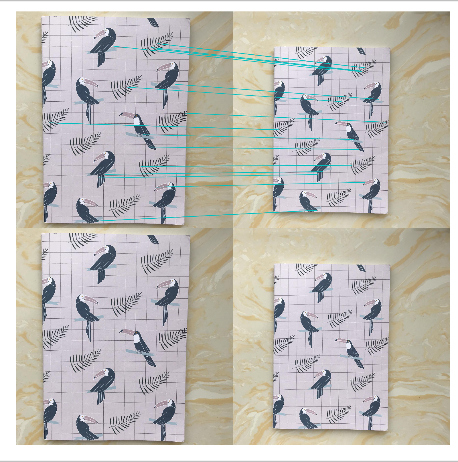实验分析：
（1）由第一组的实验结果分析可得：随着改变参数7、8、10，即匹配点的个数，得到的基础矩阵是各不相同的，对基础矩阵的有非常大的影响；除此之外匹配点的个数对于最终得到的特征匹配图也有较大的影响，其规律不是特别明显，但当参数较大时，匹配线的数量较多。
（2）远近不同的图片组经过算法匹配效果相对于远近相同的图片组效果要差一些，甚至出现了明显的错误匹配，第一组的图片匹配点较多，但是优化后所剩下的较少，第二张匹配点相对第一组较少，但是优化过后明显特征的匹配点仍被保留。
（3）sift算法本身有时候会因为匹配点附近太过相似而出现错误匹配，这个算法便能在这个基础上相对优化，避开错误的匹配，但是以上的结果都出现了一个情况，优化后的结果仍存在错误的匹配点，整体效果并不好，由于图像远近以及光线的影响，呈现的效果也不够理想，而相对的，远近相同的测试图片效果会比远近相差较大的图片要好，更容易匹配。
（4）在本次实验中，三组数据相较来说，平移变换图像相比较其他两种构图方式的图像有着较多的特征匹配数，因为平移变换对物体特征点的影响较小，而远近和左右角度变换都会在不同程度上影响到特征点的收集和遮蔽。

3.3 极点与极线

3.3.1 实验代码

import numpy as np
import cv2 as cv
from matplotlib import pyplot as plt
def drawlines(img1, img2, lines, pts1, pts2):
''' img1 - image on which we draw the epilines for the points in img2
lines - corresponding epilines '''
r, c = img1.shape
img1 = cv.cvtColor(img1, cv.COLOR_GRAY2BGR)
img2 = cv.cvtColor(img2, cv.COLOR_GRAY2BGR)
for r, pt1, pt2 in zip(lines, pts1, pts2):
color = tuple(np.random.randint(0, 255, 3).tolist())
x0, y0 = map(int, [0, -r / r])
x1, y1 = map(int, [c, -(r + r * c) / r])
img1 = cv.line(img1, (x0, y0), (x1, y1), color, 1)
img1 = cv.circle(img1, tuple(pt1), 5, color, -1)
img2 = cv.circle(img2, tuple(pt2), 5, color, -1)
return img1, img2
img1 = cv.imread('data/1.jpg', 0)  # queryimage # left image
img2 = cv.imread('data/2.jpg', 0)  # trainimage # right image
sift = cv.xfeatures2d.SIFT_create()
# find the keypoints and descriptors with SIFT
kp1, des1 = sift.detectAndCompute(img1, None)
kp2, des2 = sift.detectAndCompute(img2, None)
# FLANN parameters
FLANN_INDEX_KDTREE = 1
index_params = dict(algorithm=FLANN_INDEX_KDTREE, trees=5)
search_params = dict(checks=50)
flann = cv.FlannBasedMatcher(index_params, search_params)
matches = flann.knnMatch(des1, des2, k=2)
good = []
pts1 = []
pts2 = []
# ratio test as per Lowe's paper
for i, (m, n) in enumerate(matches):
if m.distance < 0.8 * n.distance:
good.append(m)
pts2.append(kp2[m.trainIdx].pt)
pts1.append(kp1[m.queryIdx].pt)
pts1 = np.int32(pts1)
pts2 = np.int32(pts2)
F, mask = cv.findFundamentalMat(pts1, pts2, cv.FM_LMEDS)
# We select only inlier points
# Find epilines corresponding to points in right image (second image) and
# drawing its lines on left image
lines1 = cv.computeCorrespondEpilines(pts2.reshape(-1, 1, 2), 2, F)
lines1 = lines1.reshape(-1, 3)
img5, img6 = drawlines(img1, img2, lines1, pts1, pts2)
# Find epilines corresponding to points in left image (first image) and
# drawing its lines on right image
lines2 = cv.computeCorrespondEpilines(pts1.reshape(-1, 1, 2), 1, F)
lines2 = lines2.reshape(-1, 3)
img3, img4 = drawlines(img2, img1, lines2, pts2, pts1)
plt.subplot(121), plt.imshow(img5)
plt.subplot(122), plt.imshow(img3)
plt.show()

3.3.2 实验结果及分析

① 第一组数据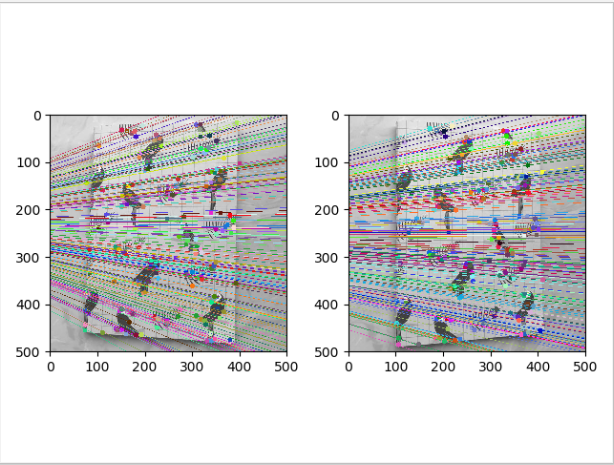② 第二组数据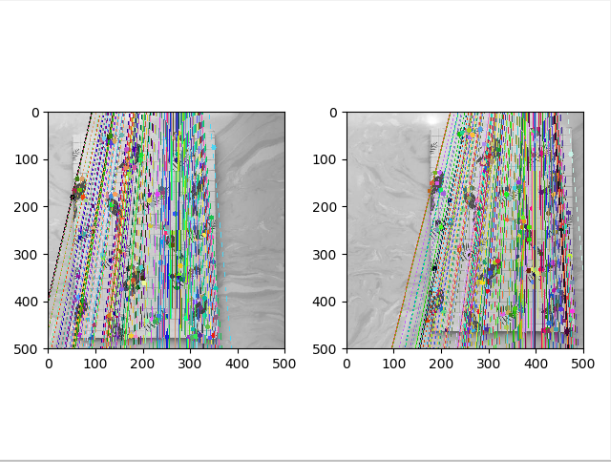③ 第三组数据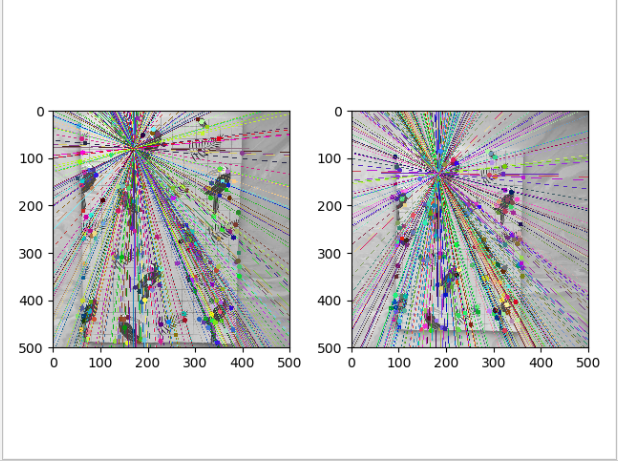实验分析：

对于第三组实验数据来说，极点为途中所有直线的交点处，由于改组图像为前后远近不同的图像组，所以该图像组的极线呈散射状，极点位于所有极线的交点处。

4. 实验中遇到的问题

在本次实验中遇到如下问题，在代码运行过程中遇到如下报错：

ImportError: cannot import name 'ransac'

解决方法：
将代码中的from PCV.geometry import ransac改为from PCV.tools import ransac即可，因为ransac.py文件夹在PCV下的tools文件夹内，不在geometry 内。

展开全文• 计算机视觉中多视几何——第一章:2D摄影几何（3.从图像恢复仿射和度量性质）

1.5 1D射影几何

直线射影几何 I P 1 IP^1 和平面射影几何 I P 2 IP^2 的推导几乎一致：

• 直线点 ： ( x 1 , x 2 ) T (x_1,x_2)^T ，用 x ˉ \bm{\bar{x}} 来表示该二维向量；
• 理想点 ： ( x 1 , 0 ) T (x_1,0)^T
• 直线的射影变换为 x ˉ ′ = H 2 × 2 x ˉ \bm{\bar{x}&#x27;} = H_{2 \times 2}\bm{\bar{x}}

其中 2 × 2 {2\times2} 齐次矩阵 H 2 × 2 H_{2\times2} 为射影变换矩阵。矩阵四元素减去一个缩放因子，所以有三个自由度。每对点提供一个约束条件，因此三组对应点可以确定直线射影变换矩阵 H 2 × 2 H_{2\times2}

交比：
交比 I P 1 IP^1 的基本射影不变量，给定4个点 x i ˉ \bm{\bar{x_i}} ,交比的定义如下：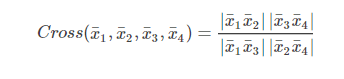其中：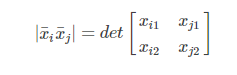交点的主要性质：

• 交比的值与个点 x i ˉ \bm{\bar{x_i}} 所用的具体齐次表示无关，因为分子分母的缩放因子将进行抵消；
• 如果每一点 x i ˉ \bm{\bar{x_i}} 都是有限点，并在齐次表示中均选择 x 2 = 1 x_2=1 ,那么 ∣ x i x j ∣ |\bm{x_i}\bm{x_j}| 就表示有 x i \bm{x_i} x j \bm{x_j} 的带符号的距离。
• 如果点 x i \bm{x_i} 中有一个理想点，交比的定义仍然有效。
• 在任何直线的射影变换下，交比的值不变：如果有 x ˉ ′ = H 2 × 2 x ˉ \bm{\bar{x}&#x27;} = H_{2 \times 2}\bm{\bar{x}} ，则：
C r o s s ( x ˉ 1 ′ , x ˉ 2 ′ , x ˉ 3 ′ , x ˉ 4 ′ ) = C r o s s ( x ˉ 1 , x ˉ 2 , x ˉ 3 , x ˉ 4 ) Cross(\bm{\bar{x}&#x27;_1},\bm{\bar{x}&#x27;_2},\bm{\bar{x}&#x27;_3},\bm{\bar{x}&#x27;_4})=Cross(\bm{\bar{x}_1},\bm{\bar{x}_2},\bm{\bar{x}_3},\bm{\bar{x}_4})

共点线： 共点线是直线上共线点的对偶。这意味着平面上的共点线也有几何 I P 1 IP^1 。特别是任何四条共点线都有一个确定的交比。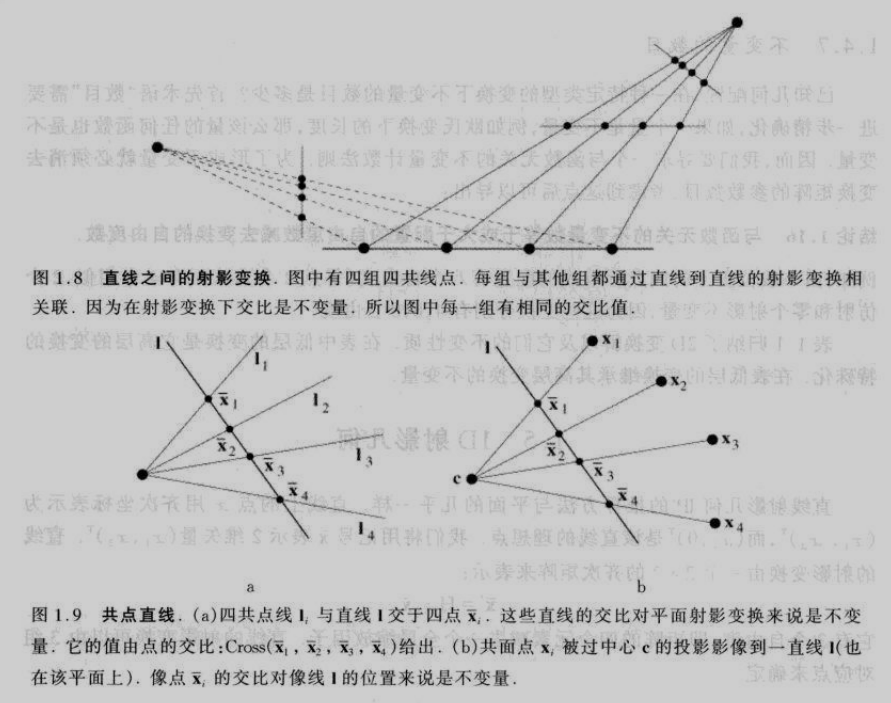由于《计算机视觉中的多视图几何》一书中在第0篇中引入了较多的概念和推导，将有利于自己对后文的理解，于是博主第0篇的笔记会写的详细一些，这样条理更清楚，也有利于后期返回来查相关概念和推导。

参考资料:

1、《计算机视觉中的多视图几何》

展开全文计算机视觉 多视图几何 射影变换
• 2017年10月23日 如此定义直线的交点的原因是，两个向量的叉积是垂直于这两个向量的，然后正好直线上的点满足aX+bY+c=0，即矩阵和向量的乘积为0，定义符合要求。计算机视觉 多视图几何 学习笔记
• 计算机视觉巨著《计算机视觉中视图几何(中文版) 》高清带书签
• 吴福朝著，全书分为三篇18章，射影几何、矩阵与张量、模型估计计算机视觉
• 一、对极几何 1.1 对极几何的概念 1.2 基本模型 1.3 对极几何约束 二、基础矩阵（F） 三、基础矩阵估计方法 3.1 八点估算法 3.2 ransac随机采样一致法估算 四、基础矩阵估计代码实现 4.1 代码 4.2 代码...计算机视觉
• Multiple View Geometry in Computer Vision( 计算机视觉中视图几何 )英文文档各一份 hartley 大神之作...